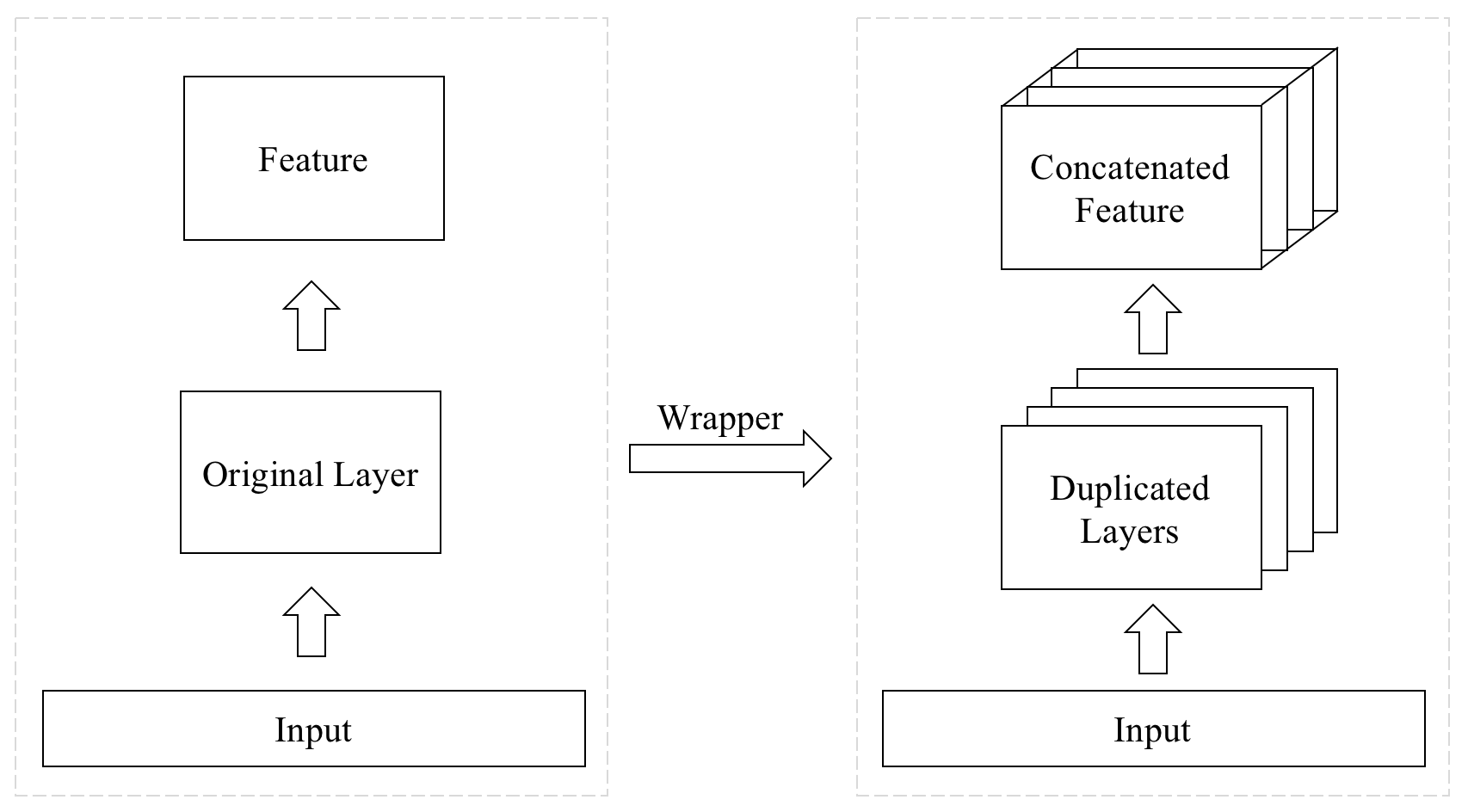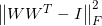Showing:

0

189

3mos ago

2

## Package

0

MIT

### CategoriesA wrapper layer for stacking layers horizontally.## Install

``````pip install keras-multi-head
``````

## Usage

### Duplicate Layers

The layer will be duplicated if only a single layer is provided. The `layer_num` argument controls how many layers will be duplicated eventually.

``````import keras

model = keras.models.Sequential()
model.build()
model.summary()
``````

### Use Multiple-Layers

The first argument could also be a list of layers with different configurations, however, they must have the same output shapes.

``````import keras

model = keras.models.Sequential()
], name='Multi-CNNs'))
model.build()
model.summary()
``````

### Linear Transformation

The input data will be mapped to different values of the same shape for each layer when `hidden_dim` is given.

### RegularizationThe regularization is used when you expect to extract different features from the parallel layers. You can customize the indices of weights in the layers, the intervals represent the parts of the weights and the factor of the regularization.

For example, the bidirectional LSTM layer has 6 weights by default, and the first 3s belong to the forward layer. The 2nd weight (recurrent kernel) in the forward layer controls the computation of gates for recurrent connections. The kernel for computing cell states lays in units x 2 to units x 3 of the recurrent kernel. We can used the regularization for the kernels:

``````import keras

model = keras.models.Sequential()
layer=keras.layers.Bidirectional(keras.layers.LSTM(units=16), name='LSTM'),
layer_num=5,
reg_index=[1, 4],
reg_slice=(slice(None, None), slice(32, 48)),
reg_factor=0.1,
))
model.build()
``````
• `reg_index`: The indices of `layer.get_weights()`, a single integer or a list of integers.
• `reg_slice`: `slice`s or a tuple of `slice`s or a list of the previous choices. If multiple indices are provided in `reg_index` and `reg_slice` is not a list, then `reg_slice` is assumed to be equal for all the indices. The whole array will be used if you leave this argument to `None`.
• `reg_factor`: The factor of the regularization, a float or a list of floats.

A more specific multi-head layer is provided (since the general one is harder to use). The layer uses scaled dot product attention layers as its sub-layers and only `head_num` is required:

``````import keras

input_layer = keras.layers.Input(
shape=(2, 3),
name='Input',
)
)(input_layer)
model = keras.models.Model(inputs=input_layer, outputs=att_layer)
model.compile(
loss='mse',
metrics={},
)
model.summary()
``````

The shapes of input and output tensors would be the same if only one layer is presented as input. The input layers will be considered as query, key and value when a list is given:

``````import keras

input_query = keras.layers.Input(
shape=(2, 3),
name='Input-Q',
)
input_key = keras.layers.Input(
shape=(4, 5),
name='Input-K',
)
input_value = keras.layers.Input(
shape=(4, 6),
name='Input-V',
)
)([input_query, input_key, input_value])
model = keras.models.Model(inputs=[input_query, input_key, input_value], outputs=att_layer)
model.compile(
loss='mse',
metrics={},
)
model.summary()
``````

## Rate & Review

Great Documentation0
Easy to Use0
Performant0
Highly Customizable0
Bleeding Edge0
Responsive Maintainers0
Poor Documentation0
Hard to Use0
Slow0
Buggy0
Abandoned0
Unwelcoming Community0
100